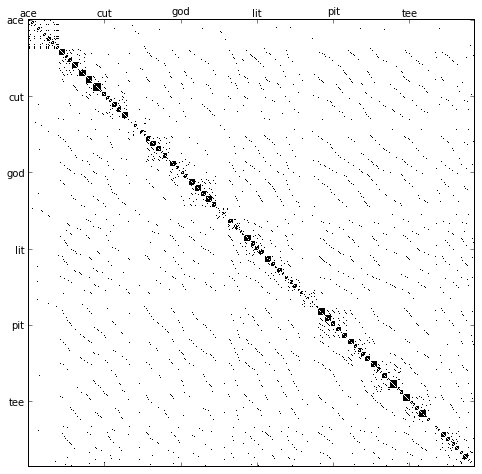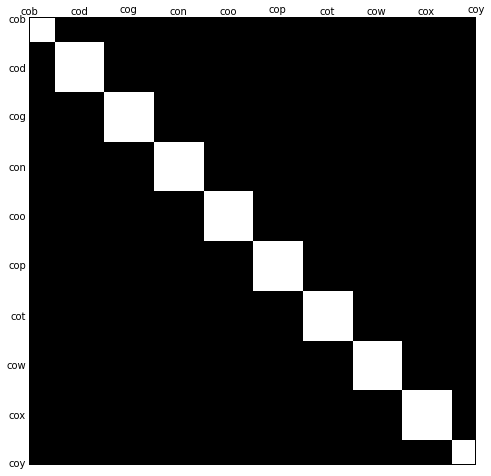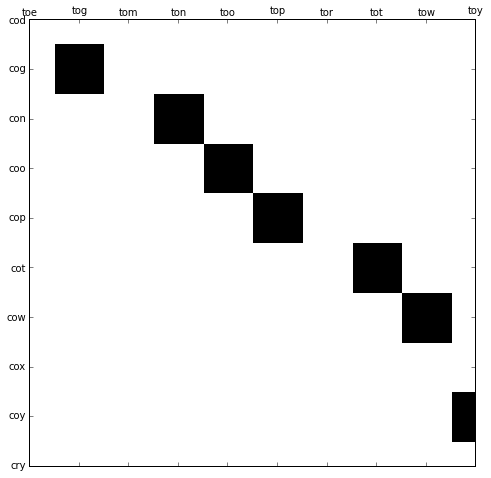# Sparse Graphs in Python: Playing with Word Ladders

The recent 0.11 release of scipy includes several new features, one of which is the sparse graph submodule which I contributed, with help from other developers. I'm pretty excited about this: there are some classic algorithms implemented, and it will open up whole new realms of computational possibilities in Python.

Before we start, I should say: this post is based on a lightning talk I gave at Scipy 2012, and some of the material below comes from a tutorial I wrote for the scipy documentation.

First: what exactly is a sparse graph? Well, a graph is just a collection of nodes, which have links between them. NetworkX has some good examples and diagrams. Graphs can represent nearly anything: social network connections, where each node is a person and is connected to acquaintences; images, where each node is a pixel and is connected to neighboring pixels; points in a high-dimensional distribution, where each node is connected to its nearest neighbors; and practically anything else you can imagine.

One very efficient way to represent graph data is in a sparse matrix: let's call it G. The matrix G is of size N x N, and G[i, j] gives the value of the connection between node i and node j. A sparse graph contains mostly zeros: that is, most nodes have only a few connections. This property turns out to be true in most cases of interest.

The creation of the sparse graph submodule was motivated by several algorithms used in scikit-learn, including:

• Isomap: a manifold learning algorithm which requires finding the shortest paths in a graph
• Hierarchical clustering: a clustering algorithm based on a minimum spanning tree
• Spectral Decomposition: a projection algorithm based on sparse graph laplacians

And many more.

Let's take a look at the package, and some of the algorithms available. Remember, this requires at least Scipy version 0.11, which was released in September 2012.

In :
# First the preliminaries: enter pylab inline mode, do some imports
%pylab inline
import numpy as np
from scipy.sparse import csgraph

Welcome to pylab, a matplotlib-based Python environment [backend: module://IPython.zmq.pylab.backend_inline].


Let's first list all the routines available in the module:

In :
csgraph.__all__

Out:
['cs_graph_components',
'connected_components',
'laplacian',
'shortest_path',
'floyd_warshall',
'dijkstra',
'bellman_ford',
'johnson',
'depth_first_order',
'depth_first_tree',
'minimum_spanning_tree',
'construct_dist_matrix',
'reconstruct_path',
'csgraph_from_dense',
'csgraph_to_dense',
'NegativeCycleError']

Lots of good stuff there! In order to show the utility of these routines, I'd like to introduce an example of a problem that can be quite interesting: word ladders.

Word ladders are a game invented by Lewis Carroll, in which words are linked by changing a single letter at each step. For example:

APE -> APT -> AIT -> BIT -> BIG -> BAG -> MAG -> MAN



Here we have gone from "APE" to "MAN" in seven steps, changing one letter each time. The question is, can we find a shorter path between these words using the same rules? As we'll see below, this problem is naturally expressed as a sparse graph problem. The nodes will correspond to individual words, and we'll create connections between words that differ by at most one letter.

### Obtaining a List of Words¶

First, of course, we must obtain a list of valid words. I'm running Ubuntu Linux, and Linux has a word dictionary at the location below. If you're on a different architecture, you may have to search a bit to find your system dictionary. But it's there somewhere...

In :
wordlist = open('/usr/share/dict/words').read().split()
len(wordlist)

Out:
99171

Our dictionary contains nearly 100,000 words. We need to reduce these to just the three-letter words, and also remove invalid words like acronyms, proper nouns, and contractions. We'll do that the following way:

In :
wordlist = filter(lambda w: len(w) == 3, wordlist) # keep 3-letter words
wordlist = filter(str.isalpha, wordlist) # no punctuation
wordlist = filter(str.islower, wordlist) # no proper nouns or acronyms

wordlist = np.sort(wordlist)
wordlist.shape

Out:
(585,)

We're left with 585 three-letter words.

Next we need to figure out how to efficiently find all pairs of words which differ by a single letter. We'll do that with some hard-core numpy type-wrangling, by converting the 3-letter words into a [585 x 3] matrix of numbers:

In :
word_bytes = np.ndarray((wordlist.size, wordlist.itemsize),
dtype='int8',
buffer=wordlist.data)
word_bytes.shape

Out:
(585, 3)

Here we've converted the raw bytes of the characters to 8-bit integers. This sort of strategy can be dangerous if you're not careful with your types, but in this instance it works fine.

### Word Ladders as a Graph¶

With our preliminary processing out of the way, we can get to the interesting part. We now have 585 points in three dimensions, and want to link all points that differ in a single dimension. One nice way to do this is to use the hamming distance in scipy: it does precisely this:

In :
from scipy.spatial.distance import pdist, squareform
from scipy import sparse

hamming_dist = pdist(word_bytes, metric='hamming')
graph = sparse.csr_matrix(squareform(hamming_dist < 1.01 / wordlist.itemsize))

graph.shape

Out:
(585, 585)

We're left with a 585 x 585 graph of our word data. To get a feeling for what this looks like, let's visualize it with matplotlib

In :
fig = plt.figure(figsize=(8, 8))
ax.matshow(graph.toarray(), cmap=plt.cm.binary)

# Label axes with the words
def formatfunc(x, *args):
if x % 1 != 0:
return ''
else:
return wordlist[max(0, min(int(x), graph.shape - 1))]

ax.xaxis.set_major_formatter(plt.FuncFormatter(formatfunc))
ax.yaxis.set_major_formatter(plt.FuncFormatter(formatfunc))There's some interesting structure in this graph! The blocks along the diagonal indicate groups of words which are closely related: for instance, "cog", "con", "coo", "cot", "cow", etc. are all near each other in alphabetical order, and share many links: this creates a dark blob on the diagonal. We can zoom in to see this:

In :
ax.set_xlim(84, 93)
ax.set_ylim(93, 84)
fig

Out:We also see linear patterns off the diagonal. These are the result of words which differ by their first letter: For example "ton" is linked to "con", "too" is linked to "coo", "top" is linked to "cop", etc. Let's zoom in and take a look at these as well:

In :
ax.set_xlim(510, 519)
ax.set_ylim(94, 85)
fig

Out:Using matplotlib's interactive plotting functionality and zooming around this graph can be a pretty interesting exercise, and help you gain further intuition into the connections between the words.

### Finding the Shortest Path: Dijkstra's Algorithm¶

Now let's return to our problem: finding the shortest path from "APE" to "MAN". This is now a graph optimization problem, in which we hope to find the shortest path from one node to another along the graph. A well-known algorithm to accomplish this task is Dyjkstra's algorithm, which is based on Dynamic Programming principles. Dijkstra's algorithm is built into the new csgraph package, and can be used as follows.

First we need to find the indices of the two words in question:

In :
i1 = wordlist.searchsorted('ape')
print(i1, wordlist[i1])

i2 = wordlist.searchsorted('man')
print(i2, wordlist[i2])

(22, 'ape')
(310, 'man')


Next we'll call the shortest_path function, which calls Dijkstra's algorithm under the hood:

In :
distances, predecessors = csgraph.shortest_path(graph, return_predecessors=True)
"distance from '%s' to '%s': %i steps" % (wordlist[i1], wordlist[i2], distances[i1, i2])

Out:
"distance from 'ape' to 'man': 5 steps"

We found a path of length 5 steps! This is shorter than the seven steps above. The steps taken are stored in the predecessor list:

In :
i = i1
while i != i2:
print(wordlist[i])
i = predecessors[i2, i]
print(wordlist[i2])

ape
apt
opt
oat
mat
man


### Finding the Longest Minimal Path¶

Another question we can ask is this: out of all the shortest paths between any two nodes, what is the longest? That is, which connected words are maximally separated in our linguistic space? To find out, we can use the distances matrix returned by the algorithm.

If any words have no path between them, the algorithm returns infinity. We don't care about the infinities here, so we'll mask them out and ask what the longest distance is:

In :
np.ma.masked_invalid(distances).max()

Out:
13.0

Evidently there exist words that cannot be linked in fewer than 13 steps! Let's see which ones they are:

In :
i1, i2 = np.where(distances == 13)
unique_paths = (i1 < i2)
zip(wordlist[i1][unique_paths], wordlist[i2][unique_paths])

Out:
[('imp', 'ohm'), ('imp', 'ohs'), ('ohm', 'ump'), ('ohs', 'ump')]

We have four pairs of words, and examining them, we see that the paths go from one pair, "imp" and "ump", to another pair, "ohm" and "ohs". We'll use the same trick as above to list the links between two of these:

In :
i = i2
while i != i1:
print(wordlist[i])
i = predecessors[i1, i]
print(wordlist[i])

ohm
oho
tho
too
moo
mod
mid
aid
ass
asp
amp
imp


### Connected Components¶

Finally, we can ask about connected components of the graph. There are likely "islands" in the word graph which can't be reached from the majority of words, and we'd like to see what these are. To do this, we can use the connected_components routine:

In :
n_components, component_list = csgraph.connected_components(graph)
n_components

Out:
14

This shows us that we have 14 distinct components: 14 islands of words with no paths between them. Let's see how big these islands are:

In :
[np.sum(component_list == i) for i in range(14)]

Out:
[571, 1, 1, 1, 2, 1, 1, 1, 1, 1, 1, 1, 1, 1]

We see that the bulk of three letter words are connected: the main set of 571 has paths to all others in that set. The interesting words are the ones all by themselves:

In :
[list(wordlist[np.where(component_list == i)]) for i in range(1, 14)]

Out:
[['aha'],
['chi'],
['ebb'],
['ems', 'emu'],
['gnu'],
['ism'],
['nth'],
['ova'],
['qua'],
['ugh'],
['ups'],
['urn'],
['use']]

These are all the three-letter "loner" words, which can't reach any other valid words through the changing of a single letter.

### More: Minimum Spanning Tree, Depth-first search, Breadth-first search...¶

There's much more we could do from here. We could use the Minimum Spanning Tree algorithm to do hierarchical clustering of the words. We could create a depth-first or breadth-first tree. We could repeat everything above on words of length 4 or length 5... if you feel inclined, check out scipy.sparse.csgraph in scipy v. 0.11!

This post was written entirely in an IPython Notebook: the notebook file is available for download here: sparse-graph.ipynb. For more information on blogging with notebooks in octopress, see my previous post on the subject.

Oh, and one challenge for all the over-achievers out there: how many steps does it take to get from "Guido" to "Python" using English language words? First to give the answer wins... well, nothing, actually. What can I say: I do this for free.

Happy coding!